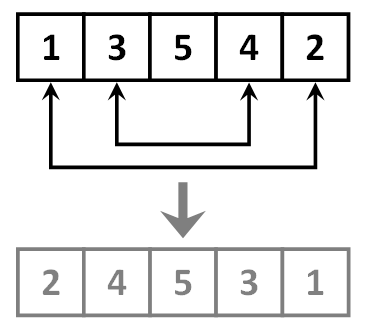# Reversing an Array

by Zoran Horvat
Dec 07, 2013

## Problem Statement

Given an array containing integer numbers, write a function which reverses the array. The first element of the array becomes the last; the second element becomes one before last, etc.

Example: Suppose that array consists of numbers 1, 3, 5, 4, 2. Function should reverse the array so that it becomes 2, 4, 5, 3, 1.

## Problem Analysis

In this exercise we need to change places of array elements. For example, first element should come to the last position in the array. However, note that last element of the array should also become the first element. Similarly, while second element should come to one before last place, the element at that place should come to the second position in the array. Conclusion is that pairs of elements should actually exchange their places, which greatly simplifies the task. Picture below shows the exchange scheme in an array with five elements:Note how the element in the middle of the array remains in place. This effect is observable only in arrays with odd length. Note also that there are only two exchange operations for the array with five elements. In six-element array, there would need to be three exchange operations. In general, reversing an array with N elements requires FLOOR(N/2) pair-wise exchange operations. Here is the function which reverses elements of an array:

``````function Reverse(a, n)
a - array with one-based indices
n - length of the array
begin
k = FLOOR(n / 2)    -- integer division
for i = 1 to k
begin
x = a[i]
a[i] = a[n - i + 1]
a[n - i + 1] = x
end
end
``````

## Implementation

Below is the complete implementation of console application in C# which generates and reverses arrays of integer numbers. Note that this implementation calculates element indices differently because arrays in C# are zero-based.

``````using System;

namespace ReversingArrays
{

public class Program
{

static int[] CreateArray(int n)
{

int[] a = new int[n];

for (int i = 0; i < n; i++)
a[i] = i + 1;

return a;

}

static void PrintArray(int[] a)
{
for (int i = 0; i < a.Length; i++)
Console.Write("{0,3}", a[i]);
Console.WriteLine();
}

static void Reverse(int[] a)
{
for (int i = 0; i < a.Length  2; i++)
{
int x = a[i];
a[i] = a[a.Length - i - 1];
a[a.Length - i - 1] = x;
}
}

static void Main(string[] args)
{

while (true)
{

Console.Write("Enter length of the array (zero to exit): ");

if (n <= 0)
break;

int[] array = CreateArray(n);

PrintArray(array);

Reverse(array);

PrintArray(array);

}

}

}
}
``````

## Demonstration

When application is run, it produces output like this:

``````Enter length of the array (zero to exit): 5
1  2  3  4  5
5  4  3  2  1
Enter length of the array (zero to exit): 6
1  2  3  4  5  6
6  5  4  3  2  1
Enter length of the array (zero to exit): 0
``````